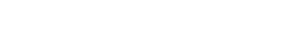# General Linear Model (GLM): Simple Definition / Overview

Probability and Statistics > Regression analysis > General Linear Model (GLM)

## What is a General Linear Model?

The General Linear Model (GLM) is a useful framework for comparing how several variables affect different continuous variables. In its simplest form, GLM is described as:

Data = Model + Error (Rutherford, 2001, p.3)

GLM is the foundation for several statistical tests, including ANOVA, ANCOVA and regression analysis. Despite their differences, each fits the definition of Data = Model + Error:

• In ANOVA, “data” is the dependent variable scores, the model is the experimental conditions, and the “error” is the part of the model not explained by the data.
• In regression analysis, the independent predictors make up the “model” and the residuals are the “error” component.
• ANCOVA is a blend of ANOVA and regression and so can also be described as Data = Model + Error.

ANCOVA is the “typical” GLM and uses at least one numerical predictor and one qualitative predictor; Some people use the term “GLM” and ANCOVA interchangeably.

## Identical ProceduresRepeated measures ANOVA is one test in the SPSS General Linear Model option.If you’re using software, the same matrix algebra equation is used for all three. They all fall under the umbrella of “GLM”, even if you find them in separate menus or procedures. If you’re in the (now unusual) situation of calculating ANOVA, ANCOVA or regression analysis by hand, time-saving computations exist for each one. This gives the illusion that they are separate entities — when in fact they are practically the same procedure.

## Formulas

If this looks familiar to the regression equation, that’s because they are one and the same. However, the key word in general linear model is general; the procedure can handle a wide variety of variables, including a non-numerical one. During the procedure, the GLM changes the non-numerical variable to a number before any calculations are made.

When the GLM βs (pronounced “betas”) are standardized with a mean of zero and a standard deviation of 1 (i.e. they are given z-scores), they are called beta weights. Otherwise, they are usually called Bs (as in the letter B in the English alphabet). The GLM equation with standardized βs is:## Emergence of the GLMM

Although many software packages still refer to certain procedures as “GLM”, the concept of a general linear model is seen by some as somewhat dated. It’s well recognized that the models can have non-linear components. There’s even some debate about the “general” part:

Calling it “general” seems quaint. It is certainly misleading ~ Stroup (2016).

Stroup prefers the term generalized linear mixed model (GLMM), of which GLM is a subtype. GLMMs combine GLMs with mixed models, which allow random effects models (GLMs only allow fixed effects). However, GLMM is a new approach:

GLMMs are still part of the statistical frontier, and not all of the answers about how to use them are known (even by experts) ~ Bolker.

Note: Don’t confuse the general linear model with the Generalized Linear Model (GLZ). GLZ is a variant of GLM which uses Bayesian hypothesis testing to predict outcomes.

## References

Bolker, B. (2017). Draft PDF posted on website: http://ms.mcmaster.ca/~bolker/classes/s4c03/notes/GLMM_Bolker_draft5.pdf
Rutherford (2001). Introducing Anova and Ancova: A GLM Approach. SAGE.
Stroup, W. (2016). Generalized Linear Mixed Models: Modern Concepts, Methods and Applications. CRC Press.

CITE THIS AS:
Stephanie Glen. "General Linear Model (GLM): Simple Definition / Overview" From StatisticsHowTo.com: Elementary Statistics for the rest of us! https://www.statisticshowto.com/general-linear-model-glm/
---------------------------------------------------------------------------Need help with a homework or test question? With Chegg Study, you can get step-by-step solutions to your questions from an expert in the field. Your first 30 minutes with a Chegg tutor is free!